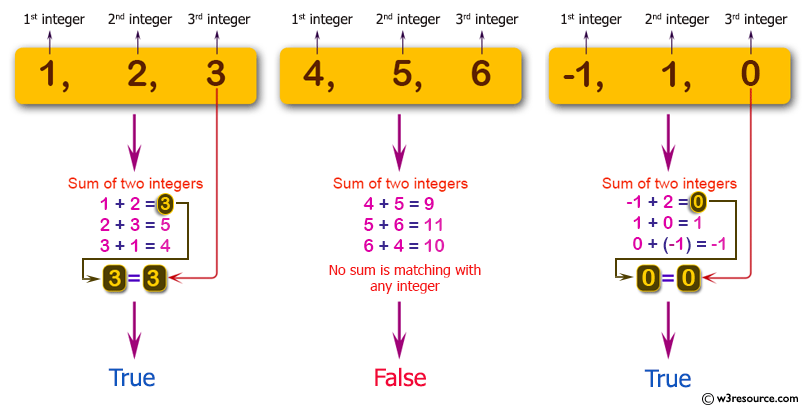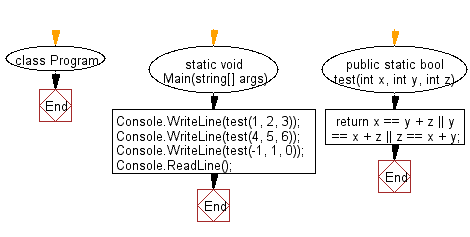﻿ C# - From three integers, add two to get the third# C# Sharp Basic Algorithm Exercises: Check whether it is possible to add two integers to get the third integer from three given integers

## C# Sharp Basic Algorithm: Exercise-47 with Solution

Write a C# Sharp program to check whether it is possible to add two integers to get the third integer from three given integers.

Pictorial Presentation:Sample Solution:-

C# Sharp Code:

``````using System;
using System.Linq;
namespace exercises
{
class Program
{
static void Main(string[] args)
{
Console.WriteLine(test(1, 2, 3));
Console.WriteLine(test(4, 5, 6));
Console.WriteLine(test(-1, 1, 0));
}
public static bool test(int x, int y, int z)
{
return x == y + z || y == x + z || z == x + y;
}
}
}
```
```

Sample Output:

```True
False
True```

Flowchart:C# Sharp Code Editor:

Improve this sample solution and post your code through Disqus

What is the difficulty level of this exercise?

Test your Programming skills with w3resource's quiz.

﻿